Pre-digital Calculating, Filing & Weather Forecasting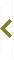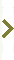# Mechanical calculating machines, mid-20th Centur

Guest contribution

There were ways to ease the task of lengthy calculations before electronic calculators and computers were commonplace. One was slide rules, another was books of tables, and another was mechanical calculators. I regularly used a mechanical calculator while working in a laboratory in the 1950s.

Tap/click for enlargements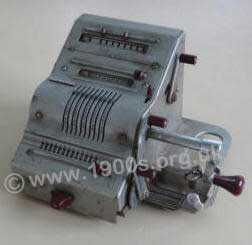Brunsviga 13RK calculating machine, 1950s.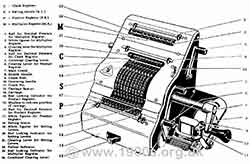Labelled parts of the Brunsviga 13RK.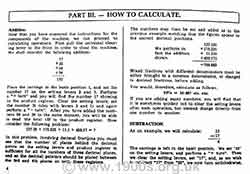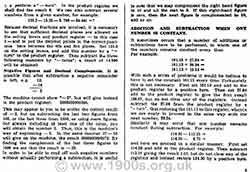Page from the manufacturer's instruction booklet showing how to subtract.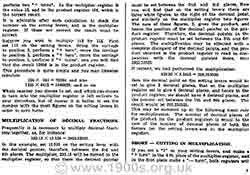Page showing how to handle fractions and decimals.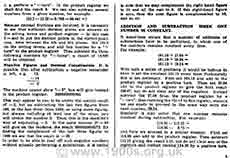More about using the Brunsviga 13RK

## Types of mechanical calculating machine

There were several makes of these mechanical calculators, and a mechanical calculator of sorts could be found in most large offices and laboratories.

The one that I used was a Brunsviga 13RK - see the photograph - which was particularly sophisticated. It is still in my possession because I rescued it when it was being thrown out.

## How mechanical calculating machines worked

What follows in this section is very much a simplification of how mechanical calculators worked, but it will serve as a basis for explaining what the calculators were like to use, which is in the next section.

At the heart of mechanical calculators were two drums made up of sets of identical discs with 0 to 9 printed round their circumference.

One disc could be set to show the first digit of the first number in a calculation, and its drum could be rotated against the second drum. This mechanical 'brushing' transferred the number on the first drum to the second drum. The transfer was by a clever use of pins sticking out of the discs on the rotating drum: 0 pins if a disc was set to 0; 1 pin if set to 1; 2 pins if set to 2 etc. The process was one of adding the number on the rotating drum to the second drum.

The machines were operated by turning handles or pulling levers. The Brunsviga 13RK worked with handles. By turning the handle slowly enough, it was possible to hear the clicks and see the number on the second drum appearing as if it was being slowly added - not that the motion needed to be slow in practice.

The rotating drum could be shifted left one disc spacing at a time which was equivalent to making the number it held 10 times larger.

A second turn of the handle added the number twice which was equivalent to multiplying it by two, three turns added it three times, which was equivalent to multiplying it by 3, etc - although there were shortcuts for multiplying large numbers. To aid reading the output, a counter showed how many times the rotating drum had turned.

Division was achieved by a form of subtraction with the handle turning the in the reverse direction.

Trust me it is very simple when you are used to it, but is easier to demonstrate than to put into words. If you would like a thorough explanation for the Brunsviga 13RK, the first few pages of the manufacturer's instruction booklet are in large legible images. Just click the small images.

The Brunsviga 13RK was capable of more than simple addition, subtraction, multiplication and division: it could also do square roots, percentages and currency conversions.

## Pros and cons of mechanical calculating machines

When you realise that back in the 1950s few people could imagine electronic calculators, the advantages of mechanical calculating machines were considerable. Slide rules were quick to use but only really accurate to two or at best three significant figures, and the most common books of tables were accurate to four significant figures. In contract, mechanical calculators were accurate to as many figures as there were rotors. In the case of the Brunsviga 13RK, this meant that a calculation could be accurate to nine significant figures.

On the negative side, though, calculations were time consuming. Remember that to multiply by nine, for example, the handle had to be turned nine times. Also as the machines contained a lot of metal and were precision-made, they were too expensive to buy in bulk. So one was not always to hand when needed because someone else was using it. Furthermore the machines were very heavy to move and were not exactly quiet in operation.

To be fair, one quickly got used to the negative aspects of these mechanical calculating machines. What we didn't know, we didn't miss. Also, for someone of the same turn of mind as me, there was a fascination about them and an admiration for how they worked.

Guest contribution

Under the disadvantages mentioned above is the large number of turns needed to multiply by 9. We used the Brunsviga calculators in technical college for a numerical analysis course in the late 1960s in the UK and were taught some shortcuts, most of which I forget now. I do remember, though, that any number over 6 was handled by left shifting to next wheel and turn once (multiply by 10) then right shift back and REVERSE wind the complement of the number e.g. once for 9, twice for 8 etc. So, to multiply by say 98, we left shifted twice and rotated once to multiply by 100 then right shifted back to the units and reverse wound twice. We could of course do the reverse wind first, then we only needed to do the shift operation once but this meant a large number of pins being moved as the full register was tripped backwards before again moving them all forwards, so it didn't really save any effort and was harder on the machine.

Greg Everard

Page contributed by Neil Cryer